SLVS949F May   2009  – May 2017

PRODUCTION DATA.

1. Features
2. Applications
3. Description
4. Revision History
5. Pin Configurations and Functions
6. Specifications
7. Detailed Description
1. 7.1 Overview
2. 7.2 Functional Block Diagram
3. 7.3 Feature Description
4. 7.4 Device Functional Modes
8. Application and Implementation
1. 8.1 Application Information
2. 8.2 Typical Application
1. 8.2.1 Design Requirements
2. 8.2.2 Detailed Design Procedures
3. 8.2.3 Application Curves
9. Power Supply Recommendations
10. 10Layout
11. 11Device and Documentation Support
12. 12Mechanical, Packaging, and Orderable Information

#### Package Options

Refer to the PDF data sheet for device specific package drawings

• RHL|14
• RGY|14

## 8 Application and Implementation

NOTE

Information in the following applications sections is not part of the TI component specification, and TI does not warrant its accuracy or completeness. TI’s customers are responsible for determining suitability of components for their purposes. Customers should validate and test their design implementation to confirm system functionality.

### 8.1 Application Information

The TPS54620 device is a highly-integrated, synchronous, step-down, DC-DC converter. This device is used to convert a higher DC input voltage to a lower DC output voltage, with a maximum output current of 6 A.

### 8.2 Typical Application

The application schematic of Figure 34 was developed to meet the requirements of the device. This circuit is available as the TPS54620EVM-374 evaluation module. The design procedure is given in this section.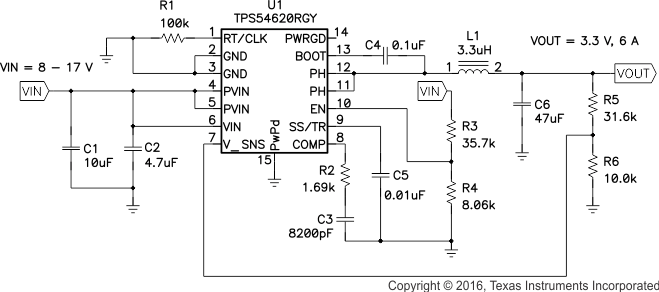Figure 34. Typical Application Circuit

#### 8.2.1 Design Requirements

This example details the design of a high frequency switching regulator design using ceramic output capacitors. A few parameters must be known to start the design process. These parameters are typically determined at the system level. For this example, the known parameters in Table 1 are used.

### Table 1. Design Parameters

DESIGN PARAMETER EXAMPLE VALUE
Output Voltage 3.3 V
Output Current 6 A
Transient Response 1A load step ΔVout = 5 %
Input Voltage 12 V nominal, 8 V to 17 V
Output Voltage Ripple 33 mV p-p
Start Input Voltage (Rising Vin) 6.528 V
Stop Input Voltage (Falling Vin) 6.190 V
Switching Frequency 480 kHz

#### 8.2.2.1 Custom Design With WEBENCH Tools

Click here to create a custom design using the TPS54620 device with the WEBENCH® Power Designer.

1. Start by entering your VIN, VOUT and IOUT requirements.
2. Optimize your design for key parameters like efficiency, footprint and cost using the optimizer dial and compare this design with other possible solutions from Texas Instruments.
3. WEBENCH Power Designer provides you with a customized schematic along with a list of materials with real time pricing and component availability.
4. In most cases, you will also be able to:
• Run electrical simulations to see important waveforms and circuit performance,
• Run thermal simulations to understand the thermal performance of your board,
• Export your customized schematic and layout into popular CAD formats,
• Print PDF reports for the design, and share your design with colleagues.

#### 8.2.2.2 Operating Frequency

The first step is to decide on a switching frequency for the regulator. There is a trade-off between higher and lower switching frequencies. Higher switching frequencies may produce a smaller solution size using lower-valued inductors and smaller output capacitors compared to a power supply that switches at a lower frequency. However, the higher switching frequency causes extra switching losses, which hurt the efficiency and thermal performance of the converter. In this design, a moderate switching frequency of 480 kHz is selected to achieve both a small solution size and a high-efficiency operation.

#### 8.2.2.3 Output Inductor Selection

To calculate the value of the output inductor, use Equation 18. KIND is a coefficient that represents the amount of inductor ripple current relative to the maximum output current. The inductor ripple current is filtered by the output capacitor. Therefore, choosing high inductor ripple currents impact the selection of the output capacitor because the output capacitor must have a ripple current rating equal to or greater than the inductor ripple current. In general, the inductor ripple value is at the discretion of the designer; however, KIND is normally from 0.1 to 0.3 for the majority of applications.

Equation 18.For this design example, use KIND = 0.3 and the inductor value is calculated to be 3.08 µH. For this design, a nearest standard value was chosen: 3.3 µH. For the output filter inductor, it is important that the RMS current and saturation current ratings not be exceeded. The RMS and peak inductor current can be found from Equation 20 and Equation 21.

Equation 19.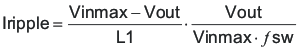Equation 20.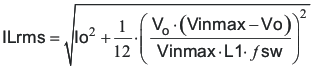Equation 21.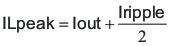For this design, the RMS inductor current is 6.02 A and the peak inductor current is 6.84 A. The chosen inductor is a Coilcraft MSS1048 series 3.3 µH. It has a saturation current rating of 7.38 A and a RMS current rating of 7.22 A.

The current flowing through the inductor is the inductor ripple current plus the output current. During power up, faults or transient load conditions, the inductor current can increase above the calculated peak inductor current level calculated above. In transient conditions, the inductor current can increase up to the switch current limit of the device. For this reason, the most conservative approach is to specify an inductor with a saturation current rating equal to or greater than the switch current limit rather than the peak inductor current.

#### 8.2.2.4 Output Capacitor Selection

There are three primary considerations for selecting the value of the output capacitor. The output capacitor determines the modulator pole, the output voltage ripple, and how the regulator responds to a large change in load current. The output capacitance must be selected based on the more stringent of these three criteria

The desired response to a large change in the load current is the first criteria. The output capacitor must supply the load with current when the regulator can not. This situation would occur if there are desired hold-up times for the regulator where the output capacitor must hold the output voltage above a certain level for a specified amount of time after the input power is removed. The regulator is also temporarily not able to supply sufficient output current if there is a large, fast increase in the current needs of the load such as a transition from no load to full load. The regulator usually needs two or more clock cycles for the control loop to see the change in load current and output voltage and adjust the duty cycle to react to the change. The output capacitor must be sized to supply the extra current to the load until the control loop responds to the load change. The output capacitance must be large enough to supply the difference in current for 2 clock cycles while only allowing a tolerable amount of droop in the output voltage. Equation 22 shows the minimum output capacitance necessary to accomplish this.

Equation 22.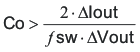where

• ΔIout is the change in output current,
• fsw is the regulators switching frequency,
• and ΔVout is the allowable change in the output voltage

For this example, the transient load response is specified as a 5% change in Vout for a load step of 1 A. For this example, ΔIout = 1.0 A and ΔVout = 0.05 × 3.3 = 0.165 V. Using these numbers gives a minimum capacitance of 25 μF. This value does not take the ESR of the output capacitor into account in the output voltage change. For ceramic capacitors, the ESR is usually small enough to ignore in this calculation.

Equation 23 calculates the minimum output capacitance needed to meet the output voltage ripple specification. In this case, the maximum output voltage ripple is 33 mV. Under this requirement, Equation 23 yields 13.2 µF.

Equation 23.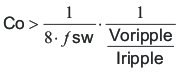where

• Vripple is the maximum allowable output voltage ripple,
• and Iripple is the inductor ripple current

Equation 24 calculates the maximum ESR an output capacitor can have to meet the output voltage ripple specification. Equation 24 indicates the ESR should be less than 19.7 mΩ. In this case, the ESR of the ceramic capacitors is much smaller than 19.7 mΩ.

Equation 24.Additional capacitance de-ratings for aging, temperature and DC bias should be factored in which increases this minimum value. For this example, a 47-μF, 6.3-V X5R ceramic capacitor with 3 mΩ of ESR is be used. Capacitors generally have limits to the amount of ripple current they can handle without failing or producing excess heat. An output capacitor that can support the inductor ripple current must be specified. Some capacitor data sheets specify the RMS (Root Mean Square) value of the maximum ripple current. Equation 25 can be used to calculate the RMS ripple current the output capacitor is required to support. For this application, Equation 25 yields 485 mA.

Equation 25.#### 8.2.2.5 Input Capacitor Selection

The TPS54620 requires a high-quality ceramic, type X5R or X7R, input decoupling capacitor of at least 4.7 µF of effective capacitance on the PVIN input voltage pins and 4.7 µF on the Vin input voltage pin. In some applications additional bulk capacitance may also be required for the PVIN input. The effective capacitance includes any DC bias effects. The voltage rating of the input capacitor must be greater than the maximum input voltage. The capacitor must also have a ripple current rating greater than the maximum input current ripple of the TPS54620. The input ripple current can be calculated using Equation 26.

Equation 26.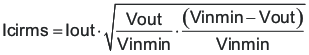The value of a ceramic capacitor varies significantly over temperature and the amount of DC bias applied to the capacitor. The capacitance variations due to temperature can be minimized by selecting a dielectric material that is stable over temperature. X5R and X7R ceramic dielectrics are usually selected for power regulator capacitors because they have a high capacitance to volume ratio and are fairly stable over temperature. The output capacitor must also be selected with the DC bias taken into account. The capacitance value of a capacitor decreases as the DC bias across a capacitor increases. For this example design, a ceramic capacitor with at least a 25-V voltage rating is required to support the maximum input voltage. For this example, one 10-μF and one 4.7-µF, 25-V capacitors in parallel have been selected as the VIN and PVIN inputs are tied together so the TPS54620 may operate from a single supply. The input capacitance value determines the input ripple voltage of the regulator. The input voltage ripple can be calculated using Equation 27. Using the design example values, Ioutmax = 6 A, Cin = 14.7 μF, Fsw = 480 kHz, yields an input voltage ripple of 213 mV and a RMS input ripple current of 2.95 A.

Equation 27.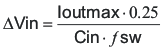#### 8.2.2.6 Slow-Start Capacitor Selection

The slow-start capacitor determines the minimum amount of time it takes for the output voltage to reach its nominal programmed value during power up. This is useful if a load requires a controlled voltage slew rate. This is also used if the output capacitance is very large and would require large amounts of current to quickly charge the capacitor to the output voltage level. The large currents necessary to charge the capacitor may make the TPS54620 reach the current limit or excessive current draw from the input power supply may cause the input voltage rail to sag. Limiting the output voltage slew rate solves both of these problems. The soft-start capacitor value can be calculated using Equation 28. For the example circuit, the soft-start time is not too critical because the output capacitor value is 47 μF, which does not require much current to charge to 3.3 V. The example circuit has the soft-start time set to an arbitrary value of 3.5 ms which requires a 10-nF capacitor. In TPS54620, Iss is 2.3 µA and Vref is 0.8 V.

Equation 28.#### 8.2.2.7 Bootstrap Capacitor Selection

A 0.1-µF ceramic capacitor must be connected between the BOOT-PH pin for proper operation. TI recommends using a ceramic capacitor with X5R or better grade dielectric. The capacitor must have a 10-V or higher voltage rating.

#### 8.2.2.8 Undervoltage Lockout Set Point

The undervoltage lockout (UVLO) can be adjusted using the external voltage divider network of R3 and R4. R3 is connected between VIN and the EN pin of the TPS54620 and R4 is connected between EN and GND . The UVLO has two thresholds, one for power up when the input voltage is rising and one for power down or brownouts when the input voltage is falling. For the example design, the supply should turn on and start switching when the input voltage increases above 6.528 V (UVLO start or enable). After the regulator starts switching, it should continue to do so until the input voltage falls below 6.190 V (UVLO stop or disable). Equation 2 and Equation 3 can be used to calculate the values for the upper and lower resistor values. For the stop voltages specified the nearest standard resistor value for R3 is 35.7 kΩ and for R4 is 8.06 kΩ.

#### 8.2.2.9 Output Voltage Feedback Resistor Selection

The resistor divider network R5 and R6 is used to set the output voltage. For the example design, 10 kΩ was selected for R6. Using Equation 29, R5 is calculated as 31.25 kΩ. The nearest standard 1% resistor is 31.6 kΩ.

Equation 29.#### 8.2.2.9.1 Minimum Output Voltage

Due to the internal design of the TPS54620, there is a minimum output voltage limit for any given input voltage. The output voltage can never be lower than the internal voltage reference of 0.8 V. Above 0.8 V, the output voltage may be limited by the minimum controllable on time. The minimum output voltage in this case is given by Equation 30

spacer

Equation 30. VOUTmin = Ontimemin × ƒsmax (VINmax + IOUTmin (RDS2min – RDS1min)) – IOUTmin (RL + RDS2min)

where

• VOUTmin = minimum achievable output voltage
• Ontimemin = minimum controllable on-time (135 ns maximum)
• ƒsmax = maximum switching frequency including tolerance
• VINmax = maximum input voltage
• IOUTmin = minimum load current
• RDS1min = minimum high-side MOSFET on-resistance (36 to 32 mΩ typical)
• RDS2min = minimum low-side MOSFET on-resistance (19 mΩ typical)
• RL = series resistance of output inductor

#### 8.2.2.10 Compensation Component Selection

There are several industry techniques used to compensate DC-DC regulators. The method presented here is easy to calculate and yields high phase margins. For most conditions, the regulator has a phase margin between 60 and 90 degrees. The method presented here ignores the effects of the slope compensation that is internal to the TPS54620. Because the slope compensation is ignored, the actual cross over frequency is usually lower than the crossover frequency used in the calculations. Use WEBENCH software for a more accurate design.

First, the modulator pole, ƒpmod, and the esr zero, ƒzmod must be calculated using Equation 31 and Equation 32. For Cout, use a derated value of 22.4 µF. use Equation 33 and Equation 34 to estimate a starting point for the closed-loop crossover frequency ƒco. Then the required compensation components may be derived. For this design example, ƒpmod is 12.9 kHz and ƒzmod is 2730 kHz. Equation 33 is the geometric mean of the modulator pole and the ESR zero and Equation 34 is the geometric mean of the modulator pole and one half the switching frequency. Use a frequency near the lower of these two values as the intended crossover frequency ƒco. In this case Equation 33 yields 175 kHz and Equation 34 yields 55.7 kHz. The lower value is 55.7 kHz. A slightly higher frequency of 60.5 kHz is chosen as the intended crossover frequency.

Equation 31.Equation 32.Equation 33.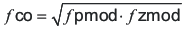Equation 34.Now the compensation components can be calculated. First calculate the value for R2 which sets the gain of the compensated network at the crossover frequency. Use Equation 35 to determine the value of R2.

Equation 35.Next calculate the value of C3. Together with R2, C3 places a compensation zero at the modulator pole frequency. Equation 36 to determine the value of C3.

Equation 36.Using Equation 35 and Equation 36 the standard values for R2 and C3 are 1.69 kΩ and 8200 pF.

An additional high frequency pole can be used if necessary by adding a capacitor in parallel with the series combination of R2 and C3. The pole frequency is given by Equation 37. This pole is not used in this design.

Equation 37.#### 8.2.2.11 Fast Transient Considerations

In applications where fast transient responses are important, the application circuit in Figure 34 can be modified as shown in Figure 35, which is a customized reference design (PMP4854-2, REV.B).

The frequency responses of Figure 35 is shown in Figure 36. The crossover frequency is pushed much higher to 118 kHz and the phase margin is about 57 degrees.

For more information about Type II and Type III frequency compensation circuits, refer to the Designing Type III Compensation for Current Mode Step-Down Converters (SLVA352) and TPS54620 & TPS54XXX Current-Mode Step-Down Converter Design Calculator (SLVC219) data sheet.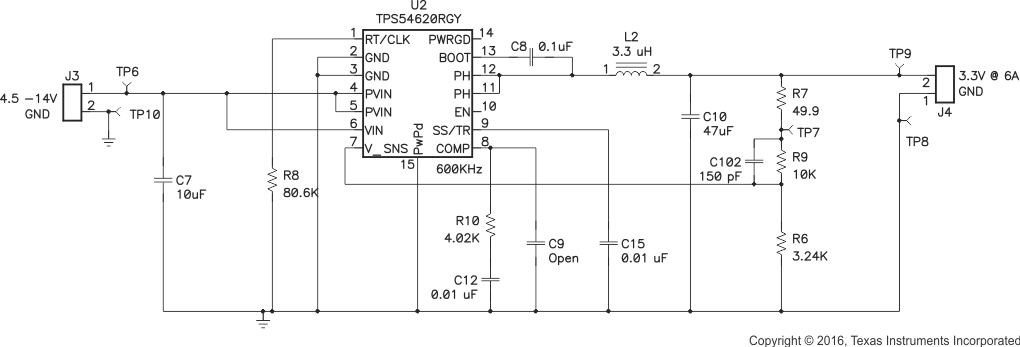Figure 35. 3.3-V Output Power Supply Design (PMP4854-2) With Fast Transients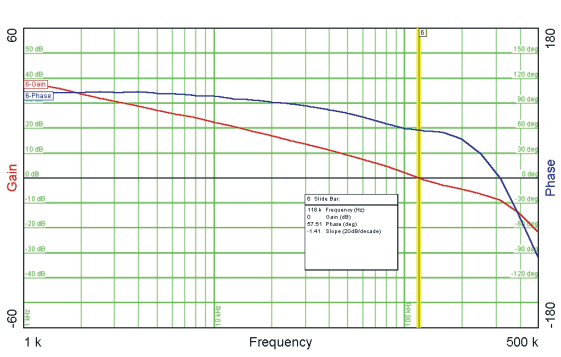Figure 36. Closed-Loop Response for PMP4854-2

#### 8.2.3 Application CurvesFigure 37. Load Transient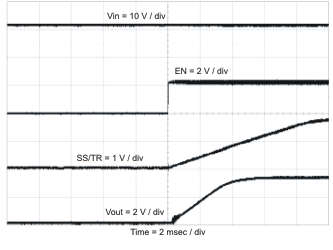Figure 39. Start-Up With ENFigure 41. Shutdown With VIN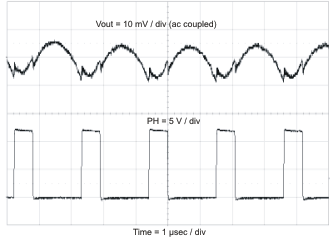Figure 43. Output Voltage Ripple With No Load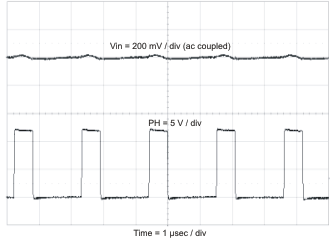Figure 45. Input Voltage Ripple With No Load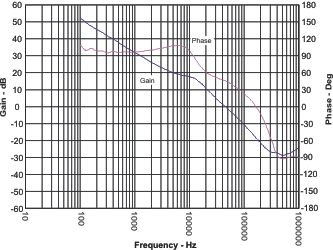Figure 47. Closed-Loop ResponseFigure 49. Load RegulationFigure 51. Maximum Ambient Temperature vs Load Current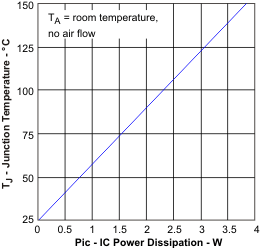Figure 53. Junction Temperature vs IC Power Dissipation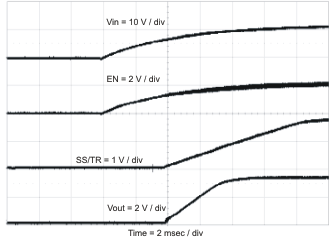Figure 38. Start-Up With VIN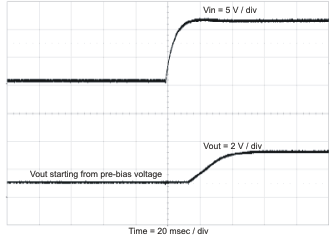Figure 40. Start-Up With PrebiasFigure 42. Shutdown With EN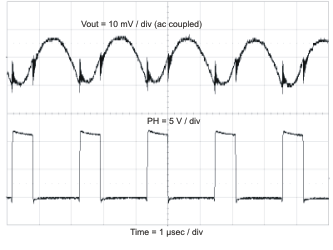Figure 44. Output Voltage Ripple With Full Load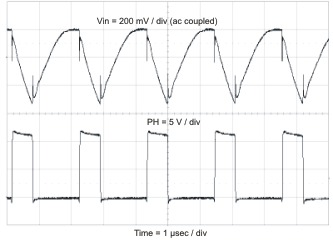Figure 46. Input Voltage Ripple With Full Load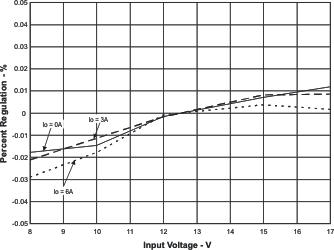Figure 48. Line RegulationFigure 50. Tracking Performance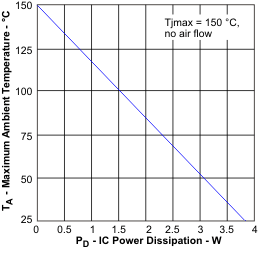Figure 52. Maximum Ambient Temperature vs IC Power DissipationFigure 54. Efficiency vs Load Current